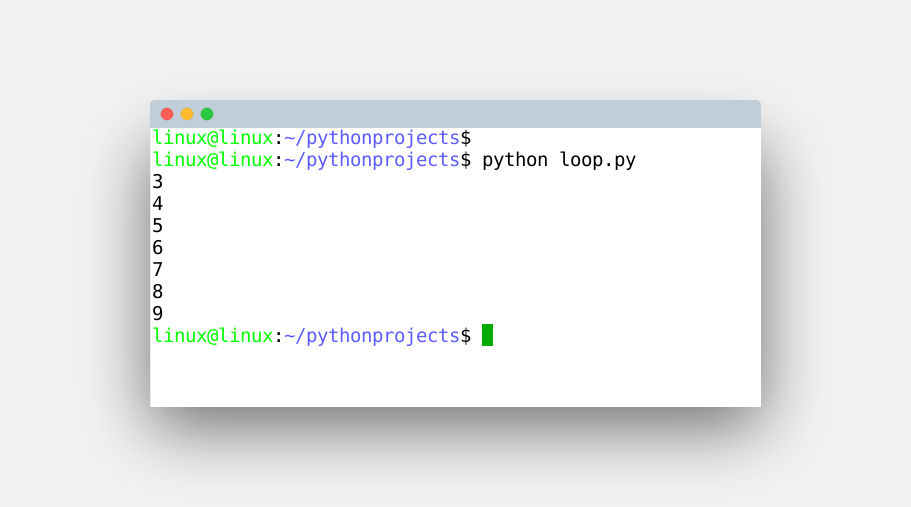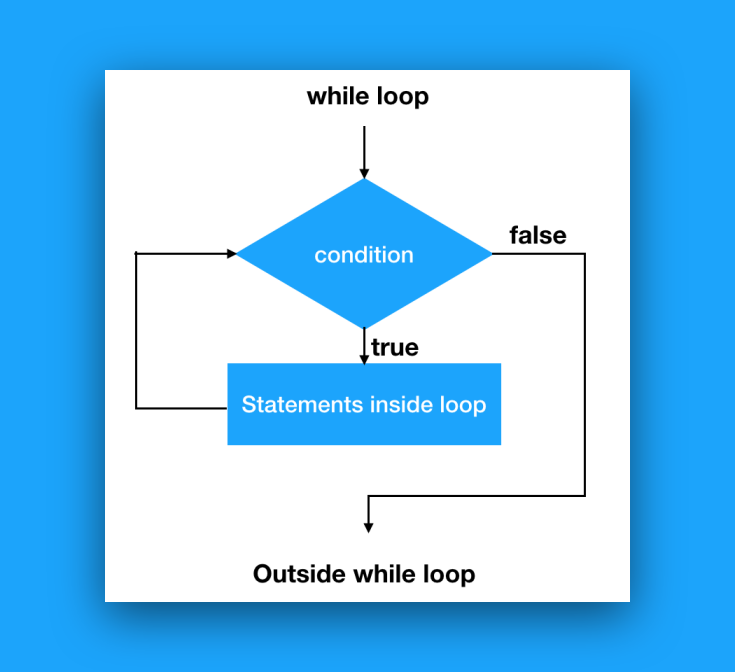A while loop repeats code until the condition is met. Unlike for loops, the number of iterations in it may be unknown. A while loop always consists of a condition and a block of code.

A while loop ends if and only if the condition is true, in contrast to a for loop that always has a finite countable number of steps.

Related course: Complete Python Programming Course & Exercises

## Example

### While loop example

The while loop below defines the condition (x < 10) and repeats the instructions until that condition is true. Type this code:

Executes the code below until the condition x < 10 is met. Unlike a for loop, the iterator i is increased in the loop.

Save then run with your Python IDE or from the terminal.You can also create infinite loops, this is when the condition never changes.

In normal cases you want the program to exit the while loop at some point. The program automatically leaves the while loop if the condition changes. Most of the times that is done with an iterator, but it could also be done by a boolean (switch).

### Control flow graph

Schematically a while loop looks like the image below. This is called the control flow graph (cfg). A visual way of what happens when a while loop is entered.

It should be noted that there can be multiple statements inside the while loop. If the code gets very long you can also call functions from inside the loop.If you are a beginner, then I highly recommend this book.

## Exercise

Try the exercise below:

1. Make a program that lists the countries in the set below using a while loop.

2. What’s the difference between a while loop and a for loop?
3. Can you sum numbers in a while loop?
4. Can a for loop be used inside a while loop?